A balun bandpass filter, very miniaturized, is demonstrated. It utilizes a combination of diagonal end-shorted coupled lines and parallel end-shorted coupled lines, both with shunt lumped capacitors instead of the λg/4 line branches of the conventional Wilkinson power divider. The proposed balun, based on that phase inverter, is included in the diagonal end-shorted coupled lines. Since these coupled lines with a lumped capacitor have a shunt resonance, the new balun can be a bandpass filter at the same time. A compact balun filter, operating at 1 GHz for experimental verification, is fabricated on a PCB substrate, with coupled lines of 15° electrical length. The die area is 3×21 mm. The balun filter achieves good amplitude and phase characteristics, as well as proper filtering performances.Figure 1 Diagonally shorted miniaturized coupled lines with shunt lumped capacitors (a) and its equivalent circuit (b).

Baluns are key components in many wireless and mobile communication systems, as well as microwave and millimeter-wave or RF circuits, such as double balanced mixers, push-pull amplifiers, frequency doublers and multipliers, in order to reduce the noise and higher-order harmonics and improve the dynamic range of the systems. In many cases, the input or output port of these baluns needs to be connected to a bandpass filter. Therefore, proper integration of the balun and bandpass filter is of practical importance to reduce the cost and size of wireless circuit systems.

Over the last few years, a lot of research has been conducted to realize the integration of the balun and bandpass filter. Some of them were based on the conventional Marchand balun with two identical coupled lines.1,2 Integrated, symmetric, four port balun bandpass filters were developed by applying specific boundary conditions.3,4 However, these balun bandpass filters were developed from the classic quarter- and half-wave resonators. Therefore, a major challenge for size reduction of balun filters still remains. Recently, a balun filter, composed of a hybrid resonant circuit employing shunt and series resonance circuits, has been developed.5 These resonators are relatively compact, λg /10 in length, where λg is the guided wavelength at the center frequency.

In this article, a balun filter for size miniaturization is introduced, using the Wilkinson power splitter structure. One side branch, which has a pair of diagonally end-shorted coupled lines and lumped capacitors, acts as a 3 λg/4 line section. The other side, which has a pair of parallel end-shorted coupled lines and lumped capacitors, is operated as a λg/4 line section. The method of adding lumped capacitors to the conventional coupled line section can largely reduce the required electrical length of a coupled line. To demonstrate its miniaturized application, a proposed balun filter was designed and fabricated. Compared with other miniaturized balun filters of different types, the realized balun bandpass filter is capable of providing a smaller size as well as maintaining good output balanced signals and useful bandpass filter.

Reduced Size Balun Filter Design Theory

Size Reduction Method of the Quarter-Wavelength Transmission Line

The quarter-wavelength transmission line has been playing a very important role in many microwave circuits. However, in many cases, it is too large to be compatible with other parts of microwave systems. The size reduction method proposed by Hirota6 has the limitation of using a high impedance transmission line in an extremely reduced size.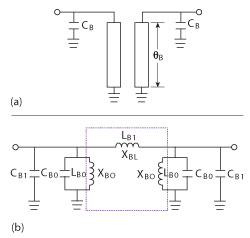Figure 2 Parallel, end shorted, miniaturized coupled lines with shunt lumped capacitors (a) and its equivalent circuit (b).

One method of improving size reduction is by replacing the high impedance section by the diagonally shorted coupled lines with shunt lumped capacitors,7 as shown in Figure 1. It is equivalent to a quarter-wave transmission line if the following equations are satisfied:where ZA, Z0, θA and ω are the characteristic impedance of the shorted coupled lines, the characteristic impedance of the quarter-wavelength line, the electrical length of the shorted line and the angular frequency, respectively. CA0 is the lumped capacitor for the miniaturization of transmission line, CA1 is the capacitor for the resonance with shunt distributed inductor and CA is the combination of CA0 and CA1. Zoe and Zoo are the even- and odd-mode characteristic impedance of the coupled lines. However, this miniaturized coupled line is actually operated as a 3 λg/4 line section, because the diagonally end-shorted coupled line section includes a phase inverter and has previously been used in a rat race coupler.8

Another method is utilizing parallel end shorted coupled lines, with shunt lumped capacitors.9 Figure 2 shows its schematic diagram (a) and its equivalent circuit (b), when Equations 3 and 4 are satisfied.Ordinary Balun Design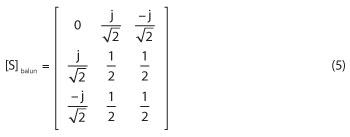Among the various kinds of baluns, a planar version of Marchand balun has been adopted for a long time, due to its various advantages. This kind of balun has been well developed.10 A block diagram of the balun is shown in Figure 3. It provides balanced outputs to load terminations Z2, from an unbalanced input with source impedance Z1. In general, the impedances Z1 and Z2 are different. When the source and load impedances are equal to Z1, it gives the best attainable S matrix of a lossless balun as follows:Figure 3 Block diagram of a symmetrical Marchand balun as two identical couplers.Figure 4 New structure for a miniaturized balun filter.

New Structure for Miniaturized Balun Filter

By employing two pairs of coupled lines instead of the λg/4 transmission line in the structure of the traditional Wilkinson power divider, a miniaturized coupled line balun is proposed (as shown in Figure 4). Since a coupled line section, grounded at the diagonal end in one branch of the modified Wilkinson power splitter balun, acts as a phase inverter, the phase difference is 180° at the output ports A and B. In addition, by comparing the baluns of figures 3 and 4, a balun with coupled lines becomes a bandpass filter with two shunt resonant circuits. Contrary to the Marchand balun, the miniaturized circuit has significant advantages, having a miniaturized balun and bandpass filter at the same time. The theoretical analysis is described as follows, in order to prove it functions as a balun.

The corresponding Y parameters of two kinds of coupled lines expressed in terms of the even- and odd-mode characteristic admittances Yoe and Yoo are given as follows:After shunting the capacitors at each side of the coupled lines, the Y parameters of two parts are:By a series of relationship about the properties of the two kinds of coupled lines deduced from Equations 1 to 4, every element of Y parameters in Equations 8 and 9 can be worked out as follows:where Y0 is the characteristic admittance of the equivalent quarter-wave transmission line of the two kinds of coupled lines. Y1 is assumed as the characteristic admittance of one branch of the modified power divider for the matching of input point of view. Then, the relationship below is satisfied:Thus, the Y parameters of the whole tri-port circuit can be derived:Based on the port terminations defined previously, Equation 15 can be converted to the scattering parameter matrix: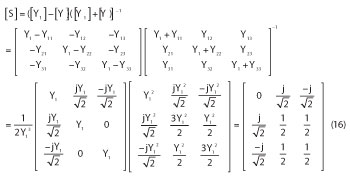This is equivalent to Equation 5, the S matrix of the ordinary Marchand balun. It is also the best attainable S-parameter matrix of a lossless balun, which is matched at the input (S11 = 0) and has transmission coefficients of -3 dB (|S21| = |S31| = (1/2)½) with opposite phase.Figure 5 Initial two-stage miniaturized balun filter model drawn in HFSS.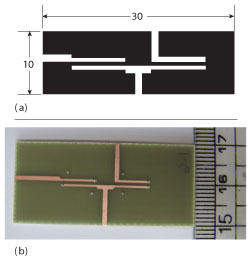Figure 6 Layout of the two-stage circuit drawn with AutoCAD (a) and its photograph (b).

Simulation and Measurement ResultsFigure 7 Simulated and measured frequency performance.

To validate the analytical results and demonstrate the design approach, the circuit parameters are converted to physical filter structures and simulated by ADS and HFSS. For the sake of simplifying the fabrication procedure as easy as can be realized, the circuit is implemented with microstrip transmission lines.

The initial miniaturized balun filter model illustrated in Figure 5, drawn in HFSS, where the electrical length of the coupled-lines being 15° at 1 GHz, was obtained. The electrical length is flexibly chosen and could be miniaturized further. When the even-mode impedance Zoe was arbitrarily chosen as 80 Ω, the value of the lumped capacitor CA and CB was calculated to be 9.68 pF, according to Equations 2 and 4 and the odd-mode impedance of the coupled-line Zoo was 50 Ω, making the coupling coefficient K = 0.23.

The layout of the balun filter drawn with AutoCAD and its photograph are shown in Figure 6. The dimensions of the circuit are 3×21 mm (not including the extended ports for testing). The width of the coupled lines is 0.65 mm, the length is 8.2 mm and the slot between the two coupled lines is 0.54 mm. The balun filter is realized on a PCB substrate having a thickness of 0.8 mm and a dielectric constant εr = 4.4. For measurement convenience, all the balanced and unbalanced ports impedances are fixed to be 50 Ω.

In Figure 7, the measured S11, S21 and S31 are compared with the simulation results from HFSS. The insertion loss for the simulated result by HFSS is 3.22 dB and the measurement result is 0.98 dB worse. The measurement performances agree well with the simulation. The additional loss is caused by surface and edge roughness of the metal, inferior metal conductivity, the dielectric loss of the substrate, and so on, which were not taken into account in the calculations.Finally, Table 1 summarizes the characteristic of several published bandpass balun filters compared with this work. The proposed bandpass filter in this article shows the advantage of more compact size and good insertion loss characteristics.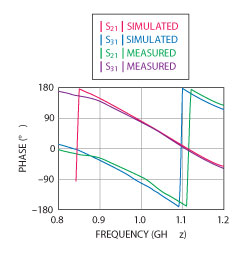Figure 8 Simulated and measured phase.

Conclusion

In this article, the design theory and procedure for a balun bandpass filter with extremely small size is carried out, utilizing the combination of diagonal shorted coupled lines and parallel end shorted coupled lines, both with shunt lumped capacitors, which offers good amplitude and phase balance performance (see Figure 8). In contrast with the conventional Marchand balun with two coupled lines, the proposed balun has the advantage that offers a miniaturized balun and a bandpass filter at the same time. In addition, this approach is theoretically able to reduce up to an extremely small size to some extent. To demonstrate the feasibility and validity of the design equation, a miniaturized balun filter, with the size of 3×21 mm, is designed and fabricated on a PCB substrate. The electrical length of the coupled lines for the fabrication could be chosen as short as 15°. The measurements agree well with the simulation results. This class of balun filter is expected to be invaluable in the design of miniaturized balanced microwave circuits.

References

1. K.S. Ang, Y.C. Leong and C.H. Lee, “Analysis and Design of Miniaturized Lumped-distributed Impedance-transforming Baluns,” IEEE Transactions on Microwave Theory and Techniques, Vol. 51, No. 3, March 2003, pp. 1009-1027.
2. R. Kravchenko, K. Markov, D. Orlenko, G. Sevskiy and P. Heide, “Implementation of a Miniaturized Lumped-distributed Balun in Balanced Filtering for Wireless Applications,” 2005 European Microwave Conference Digest, Vol. 2, pp. 1303-1306.
3. C.H. Wu, C.H. Wang and C.H. Chen, “Novel Balance Coupled-line Bandpass Filters with Common-mode Noise Suppression,” IEEE Transactions on Microwave Theory and Techniques, Vol. 55, No. 2, Part 1, February 2007, pp. 287-295.
4. C.H. Wu, C.H. Wang, S.Y. Chen and C.H. Chen, “Balanced-to-unbalanced Bandpass Filters and the Antenna Application,” IEEE Transactions on Microwave Theory and Techniques, Vol. 56, No. 11, Part 1, November 2008, pp. 2474-2482.
5. T. Yang, M. Tamura and T. Itoh, “Compact Hybrid Resonator with Series and Shunt Resonances Used in Miniaturized Filters and Balun Filters,” IEEE Transactions on Microwave Theory and Techniques, Vol. 58, No. 2, February 2010, pp. 390-402.
6. T. Hirota, “Reduced-size Branch-line and Rat-race Hybrids for Uniplanar MMIC’s,” IEEE Transactions on Microwave Theory and Techniques, Vol. 38, No. 3, March 1990, pp. 270-275.
7. I. Kang, S. Shan, X. Wang, Y. Yun, J. Kim and C. Park, “A Miniaturized GaAs MMIC Filter for the 5 GHz Band,” Microwave Journal, Vol. 50, No. 11, November 2007, pp. 88-96.
8. S.P. Marsh, “A Wide-band Stripline Hybrid Ring,” IEEE Transactions on Microwave Theory and Techniques, Vol. 16, No. 6, June 1968, pp. 361-368.
9. I. Kang and J.S. Park, “A Reduced-size Power Divider Using the Coupled Line Equivalent to a Lumped Inductor,” Microwave Journal, Vol. 46, No. 7, July 2003, pp. 72-79.
10. K.S. Ang and I.D. Robertson, “Analysis and Design of Impedance Transforming Marchand Balun,” IEEE Transactions on Microwave Theory and Techniques, Vol. 49, No. 2, February 2001, pp. 402-406.
11. T. Yang, M. Tamura and T. Itoh, “Compact and High-performance Low-temperature Co-fired Ceramic Balun Filter Using the Hybrid Resonator and Symmetric Four-port Network,” IET Microwave Antennas and Propagation, Vol. 6, No. 2, 2012, pp. 121-126.
12. C.H. Ng, E.H. Lim and K.W. Leung, “Compact Microstrip Patch Balun Filter,” IEEE 2011 Cross Strait Quad-Regional Radio Science and Wireless Technology Conference Digest, Vol. 1, pp. 1749-1751